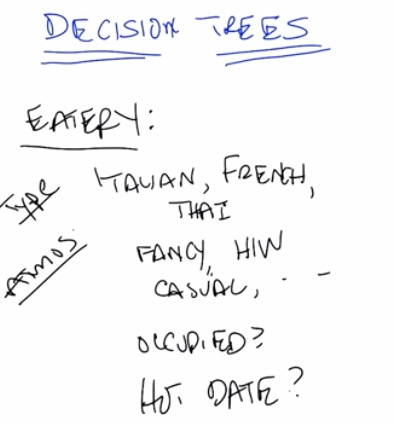• This is example for a dating problem
• Given a type,atmosphere,crowded,or a date, determine whether we want the restaurant or not. (Binary classsification problem)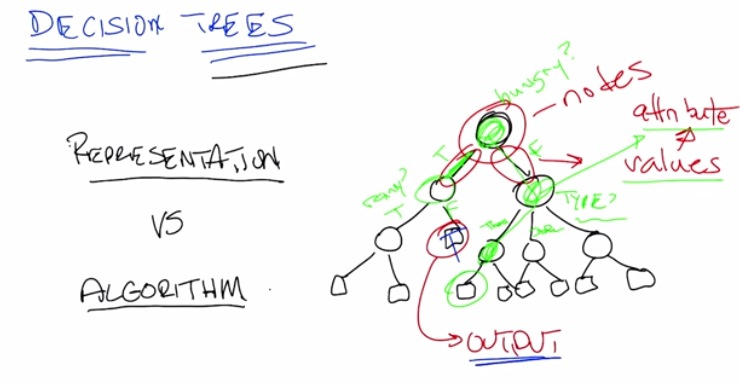• This is the decision tree.
• First step, we want to do some representation. We want to build a model for our input and determine the output
• Then we want to make an algorithm to build the model, our representation
• So representation is in our case a decision trees. We ask questions related to our features, and by giving yes/no, we move one step deeper in our tree.
• First asked hungry? Yes -> move to another node. Rainy? False, then move to the node which in turn, a leaf. The leaf said True, which means we do pick the restaurant.
• In other case, if hungry? No -> move to another side of nodes, and asked type?  then so on until we reach another leaf, that is the output.
• By then we have build a decision trees, our representation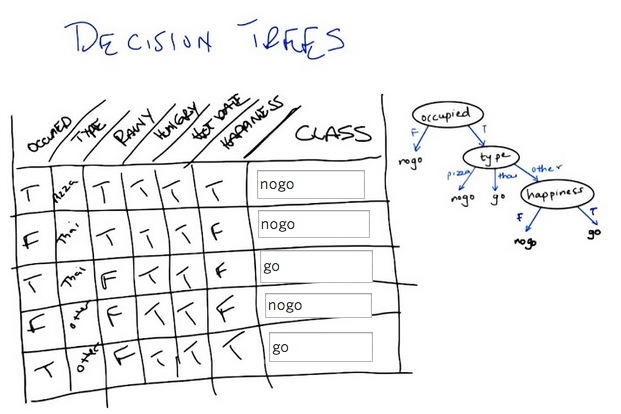• The decision tree in the left are in fact our testing set. And the one on the right is our candidate concept. By deciding the trees to only have specific trees(occupied,type,happiness) we build a decision tree just that.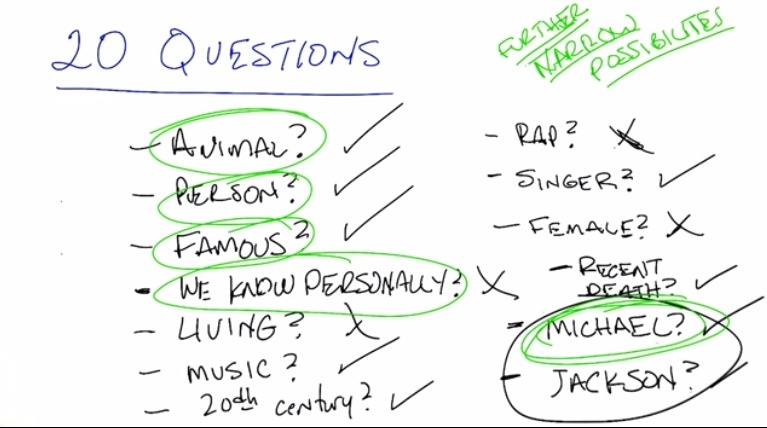• Decision Trees could be inspired by 20 Questions
• We don't know which ones we wish to sort our question in nodes, so lets take a look with the game.
• By A in thinking of Michael Jackson, B try to provide some questions to A, and A said yes/no
• We don't want to ask "Michael?". If said yes, it will give us something, but if no, then there will be harder because we don't get anything useful.
• By sorting the question, we want to take yes/no as informative and keep asking questions that narrowed it downs to the possible answer.
• This is some kind of work for decision tree.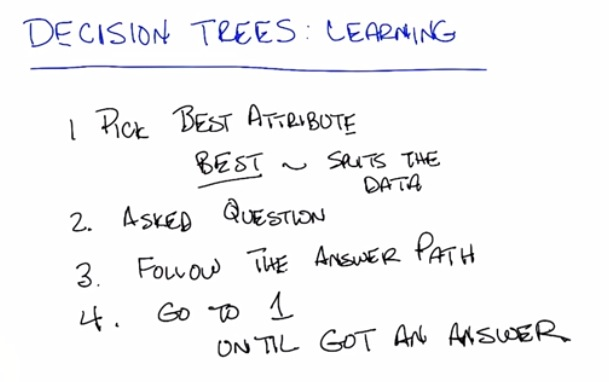• So what's the algorithm?
• First, pick best atribute. Best by spliting the category roughly in half
• Keep doing that until we got an answer
• The difference from the game(20 Questions), is we don't guess. But rather list of all the possible answers.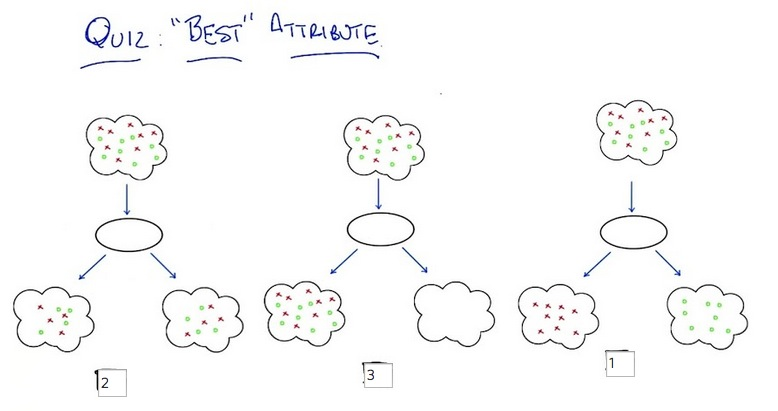• this is ranked based on the order of best
• Third is best
• Second is the worst as it done nothing to split
• First could be the worst also, only separate the groups but still doing nothing(example of overfitting). Nothing useful as to split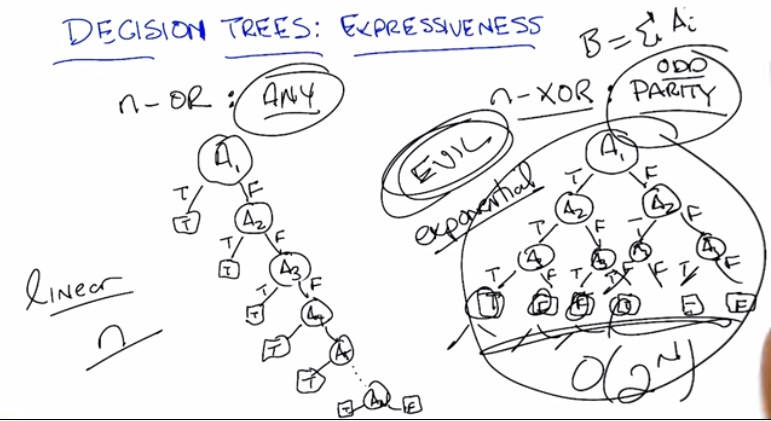• So the decision tree could be any (linear n) or ordo parity(2^n)
• this left side could be easier, but the right one is not, a lot harder
• This a two representation model, and in machine learning, it's a lot better to pick best representation for our model, or we can end up with right representation, that could be bigger exponentially as nodes increases.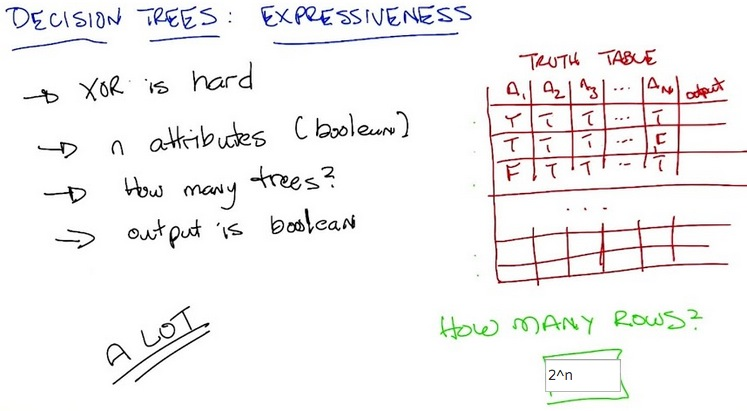• 1 node = 2 leave. 2 nodes = 4 leave, 3 nodes = 8 leaves, till it output roughly 2^n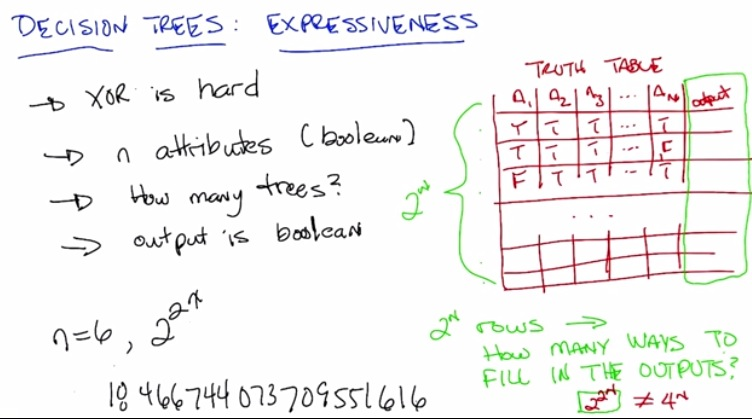• output as it a boolean, work like a bit. if bit itself depends of number position(which in case 2^n). and bit itself is 2, then the result is as above.
• Even n equals 6 will generate huge number of value.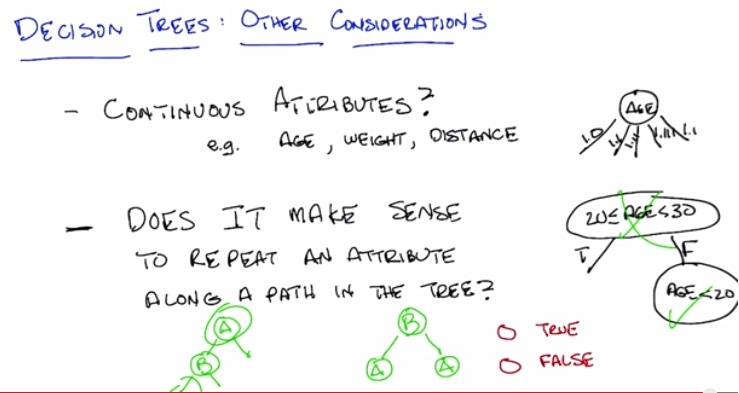• Earlier until now we have discussed is an atrribute that always discrete.
• But it might be the case if it continuous. If that's the case, we might want to range the attribute, (e.g. age). Then it does makes sense if we asking same attribute on the node, but with different kinds of range.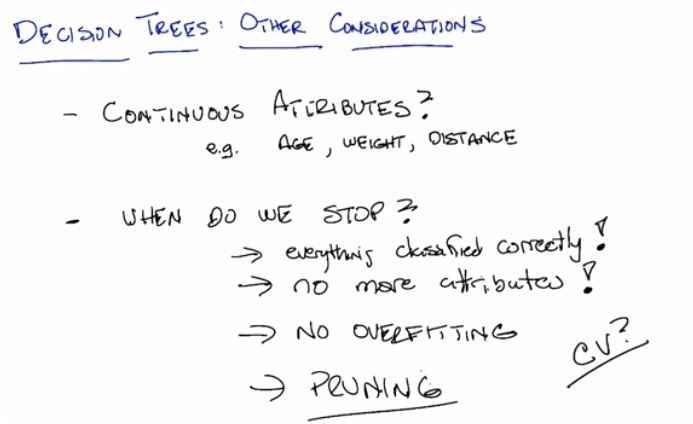• Want a perfect classification algorithm
• Keep in mind, noise must be accountable (bad input, etc)
• We wouldn't want the next instances we predict wrong output (high error rate)
• This would be the case of overfitting. As we want to generalize our algorithm to handle all data, including the noise.
• We could do take the algorithm to the cross-validation set. Take order of polynomial of each atribute or learning rate, choose the model (decision tree) with the lowest cross-validation error. And by doing this, to make it more efficient(don't just make whole tree), we expand breadth-first (rather than depth-first) and directly test in cv set.
• Or could do some pruning, take the earlier solution, and prune the attribute that doesn't do good job splitting (pruning the nodes).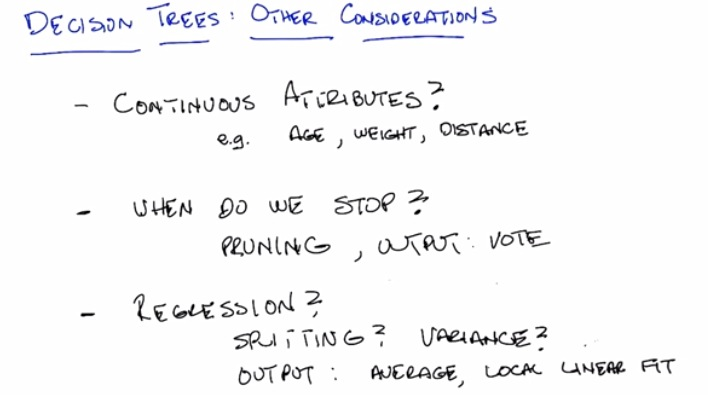• Decision trees with continous output(regression), then here's what we should do.
• We could make a vote(largest same output, labeled that), average(in a way, same as vote).
• Keep attention to variance, does it perform a good job at splitting.